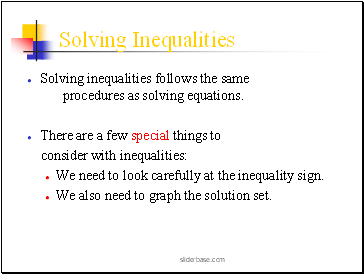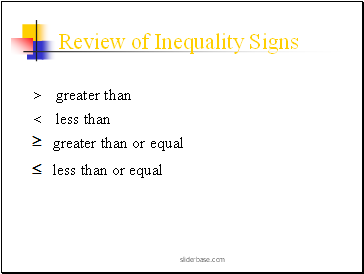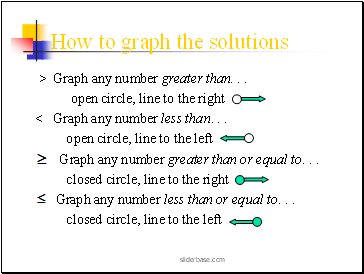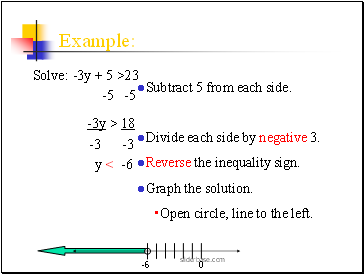# Solving InequalitiesPage 1

#### WATCH ALL SLIDES

Slide 11.6 Solving Inequalities

Slide 2## Solving Inequalities

Solving inequalities follows the same procedures as solving equations.

There are a few special things to

consider with inequalities:

We need to look carefully at the inequality sign.

We also need to graph the solution set.

Slide 3## Review of Inequality Signs

> greater than

< less than

greater than or equal

less than or equal

Slide 4## How to graph the solutions

> Graph any number greater than. . .

open circle, line to the right

< Graph any number less than. . .

open circle, line to the left

Graph any number greater than or equal to. . .

closed circle, line to the right

Graph any number less than or equal to. . .

closed circle, line to the left

Slide 5## Solve the inequality:

x + 4 < 7

-4 -4

x < 3

Subtract 4 from each side.

Keep the same inequality sign.

Graph the solution.

Open circle, line to the left.

Slide 6## There is one special case.

Sometimes you may have to reverse the direction of the inequality sign!!

That only happens when you

multiply or divide both sides of the inequality by a negative number.

Slide 7Example:

Solve: -3y + 5 >23

-5 -5

-3y > 18

-3 -3

y < -6

Subtract 5 from each side.

Divide each side by negative 3.

Reverse the inequality sign.

Graph the solution.

Open circle, line to the left.

Slide 8Try these:

Solve 2x+3>x+5

Solve - c - 11>23

Solve 3(r-2)<2r+4